# Algebra 1 : How to divide integers

## Example Questions

1 3 Next →

### Example Question #2481 : Algebra 1

Divide the following numbers: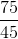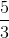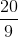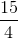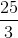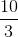Explanation:

Both numbers are divisible by.

Rewrite the numbers by their common factors.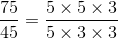The common terms that can be cancelled are three and five.

Reduce the fraction.

The answer is:### Example Question #21 : Real Numbers

Divide the following integers: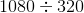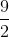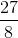Explanation:

Rewrite the expression as a fraction.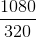Cancel out the zeros on the ones place.  This is the same as dividing both numbers by ten.

The result is: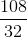Both numbers are divisible by two.   Write the fractions in terms of their common factors.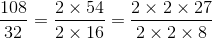The common twos can be cancelled on the numerator and denominator.

The answer is:### Example Question #23 : Integer Operations

Divide the following integers: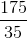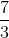Explanation:

Write the fraction using common factors.  Both are divisible by five.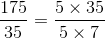Simplify the fraction.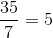This fraction reduces to a whole number.

The answer is:### Example Question #24 : Integer Operations

Divide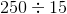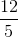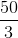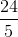Explanation:

Rewrite this expression as a fraction.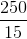Rewrite the numerator and denominator using common factors.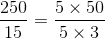Notice that we can now cancel the five in the numerator and denominator.

The answer is:### Example Question #25 : Integer Operations

Divide eighty four with sixteen.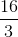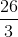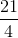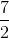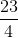Explanation:

Write the problem statement with an expression as a fraction.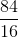Rewrite the numerator and denominator by common factors.  Both numbers are divisible by four.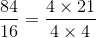Notice that we can cancel the common fours on the top and bottom.

The answer is:### Example Question #22 : Real Numbers

Divide eighty six with thirty two.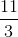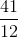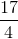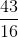Explanation:

Write the expression for this problem.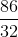Rewrite the fraction using common factors.  Both the numerator and denominator are divisible by two.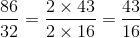This fraction is no longer reducible.

The answer is:### Example Question #2482 : Algebra 1

Divide: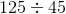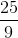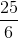Explanation:

To divide these numbers, we can rewrite this as a fraction to avoid long division.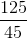Both numbers can be seen divisible by five.  Rewrite these numbers using common factors.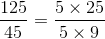Cancel the fives.

The answer is:1 3 Next →

### All Algebra 1 Resources